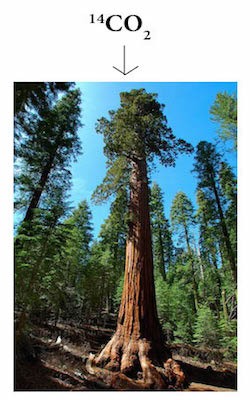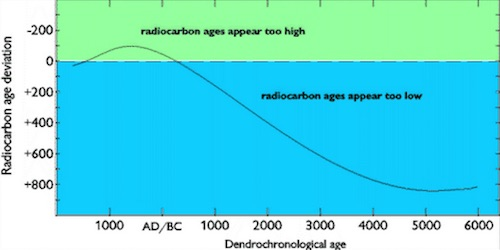# Carbon DatingCarbon Dating
Carbon Dating refers to a technique for estimating the age of dead organisms.  As depicted in the image above, the technique is based on the fact that a certain amount of carbon circulates in the atmosphere as radioactive${}^{\text{14}}\text{C}{{\text{O}}_{\text{2}}}$ , rather than as “normal” nonradioactive${}^{\text{12}}\text{C}{{\text{O}}_{\text{2}}}$ , before it is absorbed by plants for photosynthesis.  When the plant dies, the radioactive${}^{\text{14}}\text{C}{{\text{O}}_{\text{2}}}$ gradually decays over time to become nonradioactive${}^{\text{12}}\text{C}{{\text{O}}_{\text{2}}}$ .
This carbon-decaying process can take thousands of years.  By measuring the amount of${}^{\text{14}}\text{C}{{\text{O}}_{\text{2}}}$ in a dead organism (a plant or an animal that has eaten and incorporated plant nutrients into its skeleton), it is possible to estimate the age (carbon date) of a dead organism.

Dendrochronology (Tree-Ring Dating)
Dendrochronology refers to a technique for estimating the age of a tree by counting the number of rings in a horizontal cross-section of a tree trunk.  (A tree grows at a rate of one ring per year.)  As depicted in the image of a sequoia trunk below, dendrochronology can be used to estimate the age of a tree that has lived for thousands of years.Carbon Dating vs. Dendrochronology
Carbon dating is not as accurate as dendrochronology.  Over thousands of years, as depicted in the image below, carbon dating tends to underestimate age.  (Dates above zero overestimate tree-ring age; dates below zero underestimate tree-ring age.)  Changes in Earth’s climate and/or magnetic field have been postulated as reasons for this discrepancy.Practice – Questions

1.  Carbon Dating refers to a technique for estimating the age of:
A.  young people
B.  old people
C.  living organisms

2.  Carbon Dating is based on the radioactive decay of:
A.  sugar
B.  cholesterol
C.${}^{\text{14}}\text{C}{{\text{O}}_{\text{2}}}$
D.${}^{\text{12}}\text{C}{{\text{O}}_{\text{2}}}$

3. Dendrochronology refers to a technique for estimating the age of a:
A.  person
B.  car
C.  tree

4.  Over thousands of years, carbon dating tends to:
A.  overestimate age
B.  underestimate age
C.  equalize age
D.  engage in ageism

5.  Over short periods of time (less than a hundred years), carbon dating tends to:
A.  overestimate age
B.  underestimate age
C.  agree with dendrochronology
D.  shake it off

2.  C.${}^{\text{14}}\text{C}{{\text{O}}_{\text{2}}}$